# Recursion and Induction Themes Recursion Recurrence Definitions Recursive

• Slides: 49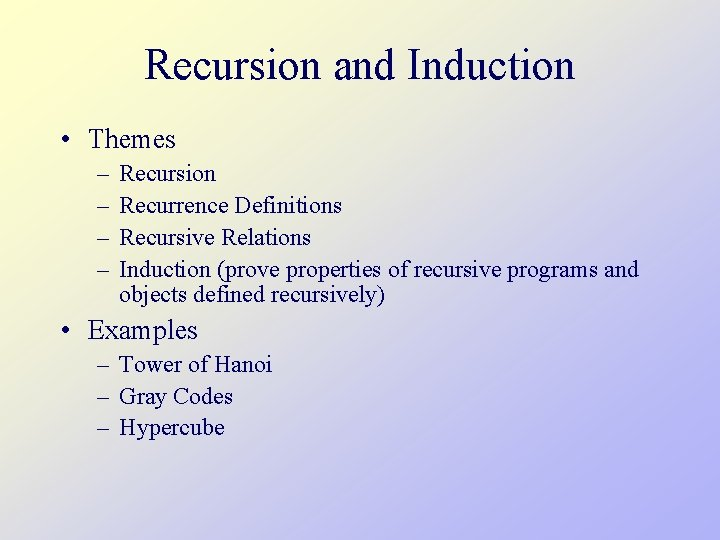Recursion and Induction • Themes – – Recursion Recurrence Definitions Recursive Relations Induction (prove properties of recursive programs and objects defined recursively) • Examples – Tower of Hanoi – Gray Codes – HypercubeRecursion & Recurrence Relations • Very handy for defining functions and data types simply: – Consider the nth Fibonacci number, Fn: • = 1, if n = 0 or n=1 • = Fn-1 + Fn-2 , for all n>1 • Very handy when a large problem can be broken in similar (but smaller) problems – We’ll look at the Towers of Hanoi in a momentWho needs Induction? This chapter will provide us with some tools, to reason precisely about algorithms, and programs – Check for correctness • Does the program end? • Does it do its job? – Check performance • How does the runtime of a particular algorithm grow vs. the inputs (number and/or size)?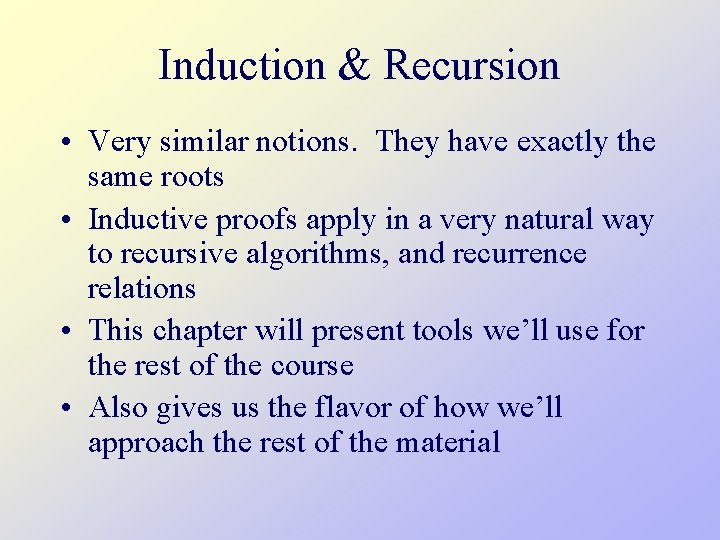Induction & Recursion • Very similar notions. They have exactly the same roots • Inductive proofs apply in a very natural way to recursive algorithms, and recurrence relations • This chapter will present tools we’ll use for the rest of the course • Also gives us the flavor of how we’ll approach the rest of the material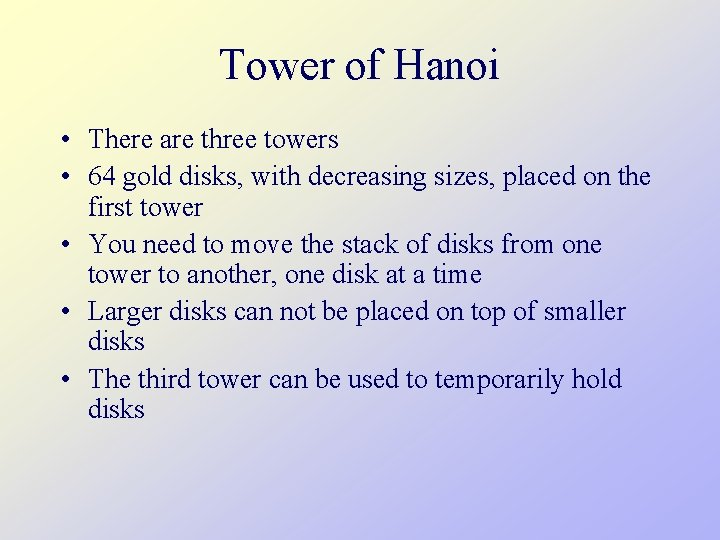Tower of Hanoi • There are three towers • 64 gold disks, with decreasing sizes, placed on the first tower • You need to move the stack of disks from one tower to another, one disk at a time • Larger disks can not be placed on top of smaller disks • The third tower can be used to temporarily hold disksTower of Hanoi • Assume one disk can be moved in 1 second How long would it take to move 64 disks? N disks? • To create an algorithm to solve this problem, it is convenient to generalize the problem to the “N-disk” problem, where in our case N = 64.Recursive SolutionRecursive Solution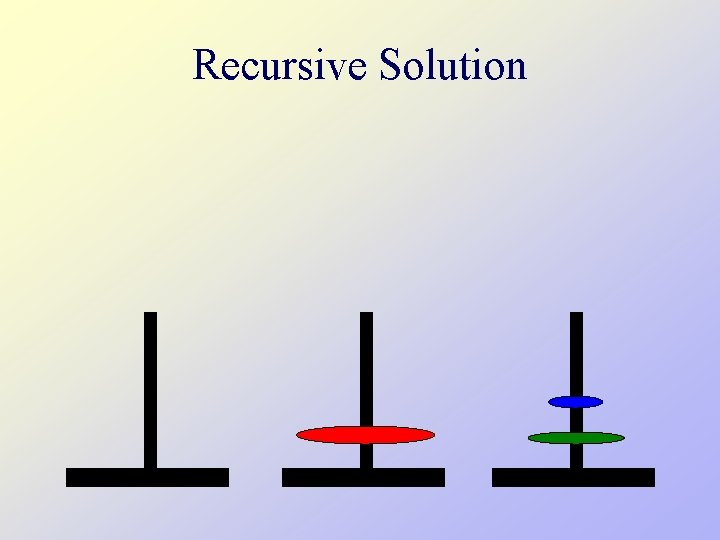Recursive Solution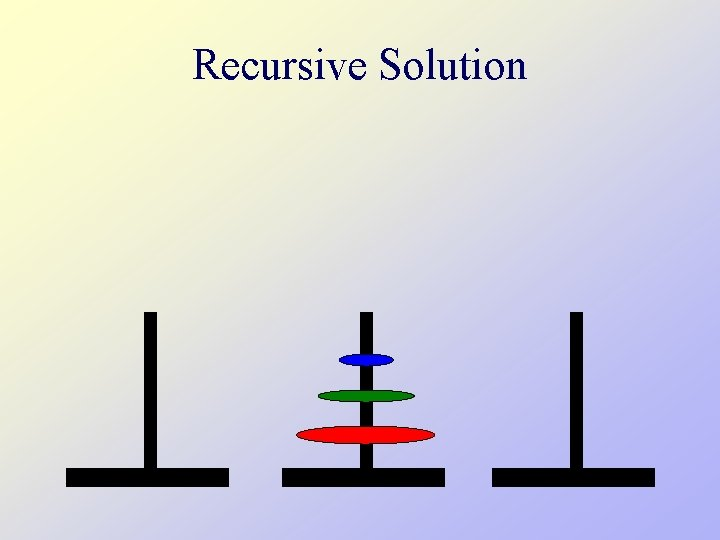Recursive Solution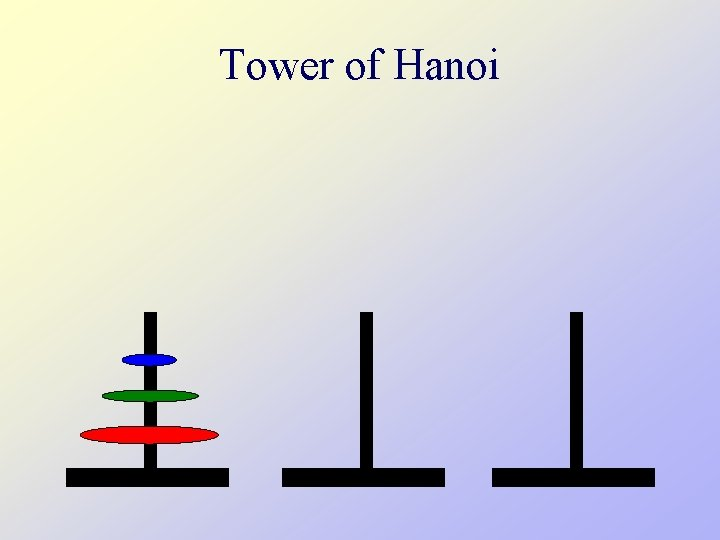Tower of Hanoi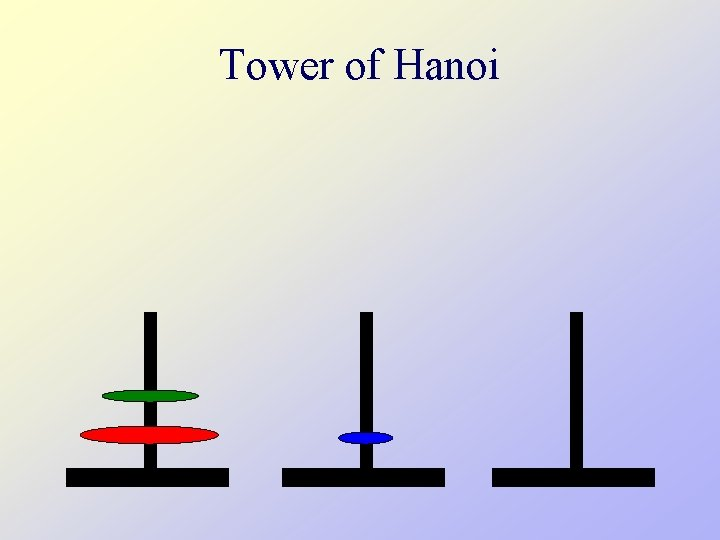Tower of HanoiTower of HanoiTower of HanoiTower of HanoiTower of Hanoi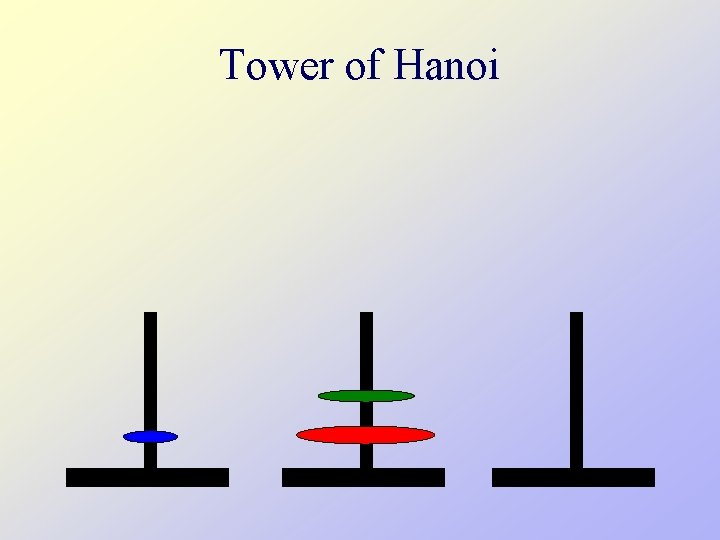Tower of Hanoi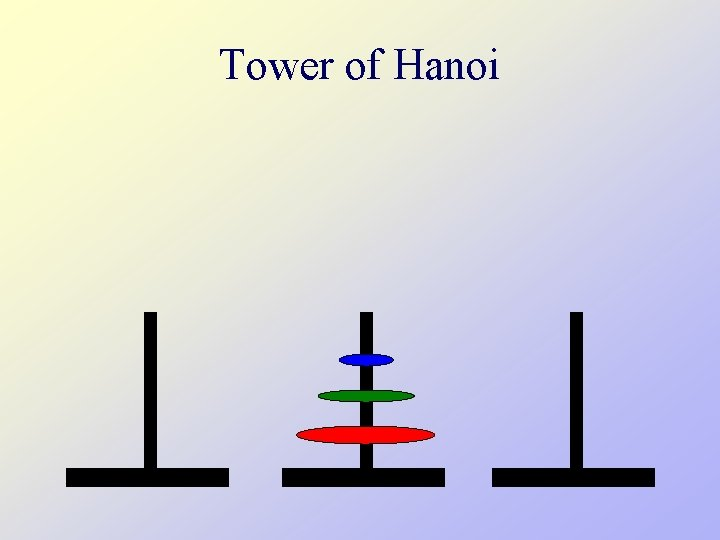Tower of Hanoi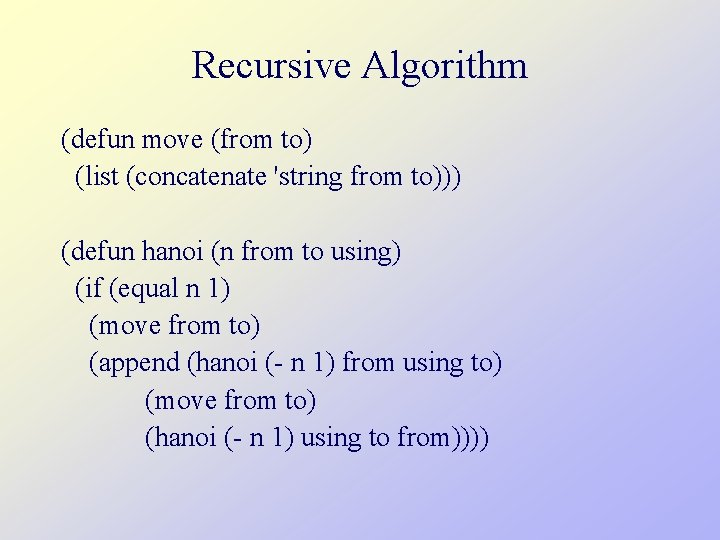Recursive Algorithm (defun move (from to) (list (concatenate 'string from to))) (defun hanoi (n from to using) (if (equal n 1) (move from to) (append (hanoi (- n 1) from using to) (move from to) (hanoi (- n 1) using to from))))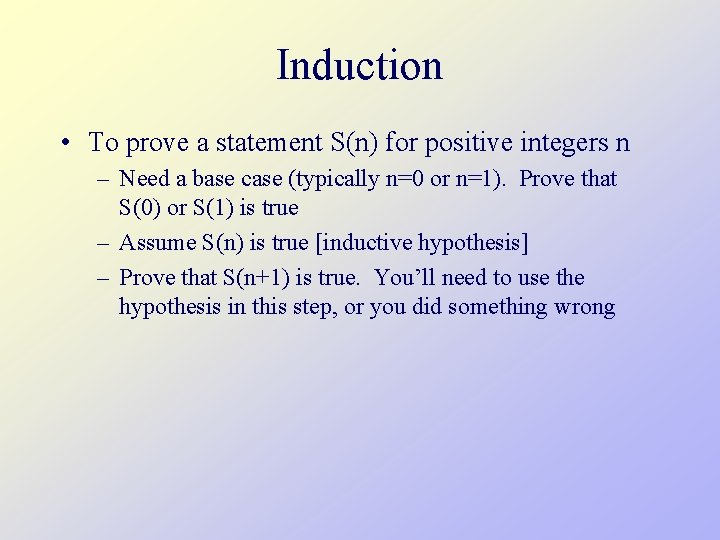Induction • To prove a statement S(n) for positive integers n – Need a base case (typically n=0 or n=1). Prove that S(0) or S(1) is true – Assume S(n) is true [inductive hypothesis] – Prove that S(n+1) is true. You’ll need to use the hypothesis in this step, or you did something wrong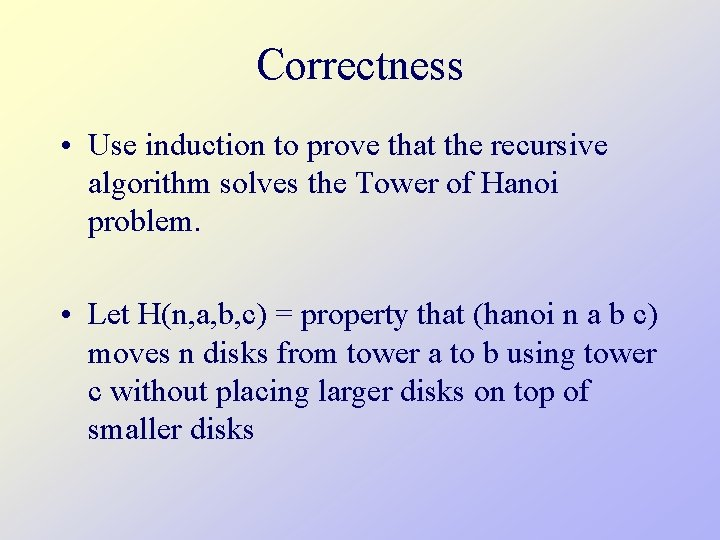Correctness • Use induction to prove that the recursive algorithm solves the Tower of Hanoi problem. • Let H(n, a, b, c) = property that (hanoi n a b c) moves n disks from tower a to b using tower c without placing larger disks on top of smaller disksCorrectness • Base case: • H(1, a, b, c) works since (hanoi 1 a b c) = (move a b) • Inductive Hypothesis (IH): • Assume H(n, a, b, c) is correct for arbitrary a b c • Show IH H(n+1, a, b, c) • The first recursive call correctly moves n disks from tower a to tower c [by IH] • Move moves the largest disk to the empty tower b (all other disks on tower c) • The second recursive call correctly moves n disks from tower c to tower b on top of largest diskCost • Show that the number of moves M(n) required by the algorithm to solve the n-disk problem satisfies the recurrence relation – M(n) = 2 M(n-1) + 1 – M(1) = 1 • This can be done inductively, and it would be very similar to the last proof.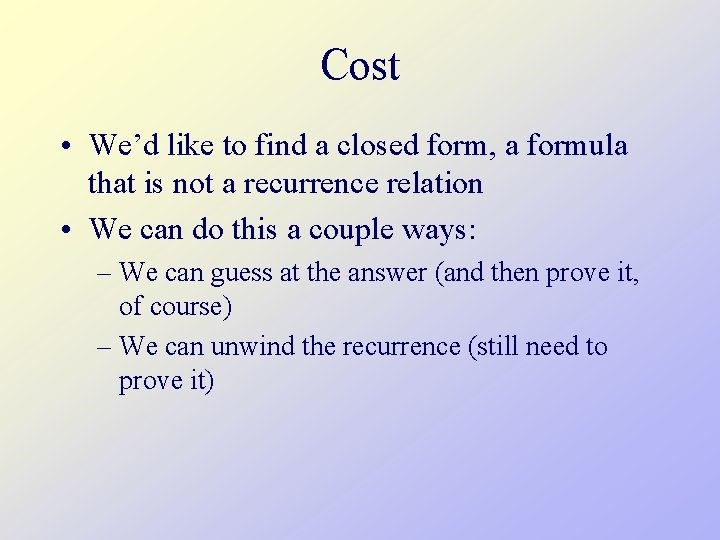Cost • We’d like to find a closed form, a formula that is not a recurrence relation • We can do this a couple ways: – We can guess at the answer (and then prove it, of course) – We can unwind the recurrence (still need to prove it)Guess and Prove • Calculate M(n) for small n and look for a pattern. • Guess the result and prove your guess correct using induction. n M(n) 1 1 2 3 3 7 4 15 5 31Proof by Induction • Base case: M(1) = 1 = 21 -1 • Inductive Hypothesis (IH) • Assume M(n) = 2 n-1 • Show IH M(n+1) = 2 n+1 -1 • M(n+1) = 2 M(n) + 1 • = 2(2 n-1) + 1 [by IH] • = 2× 2 n -1= 2 n+1 -1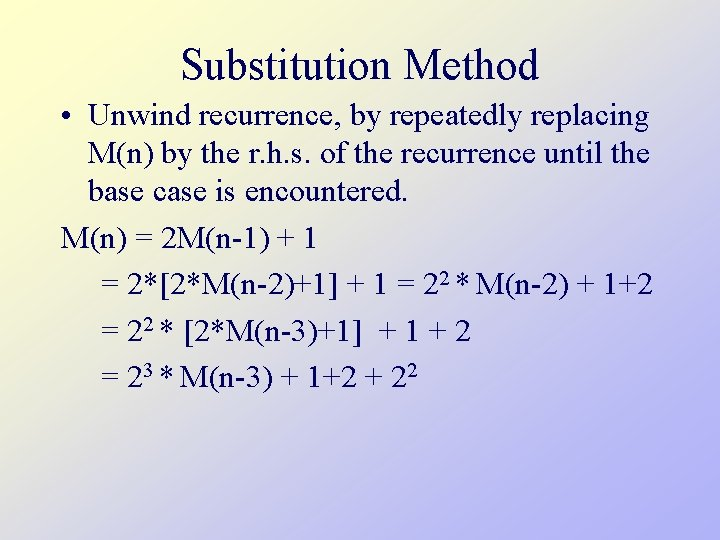Substitution Method • Unwind recurrence, by repeatedly replacing M(n) by the r. h. s. of the recurrence until the base case is encountered. M(n) = 2 M(n-1) + 1 = 2*[2*M(n-2)+1] + 1 = 22 * M(n-2) + 1+2 = 22 * [2*M(n-3)+1] + 1 + 2 = 23 * M(n-3) + 1+2 + 22Geometric Series • After k steps: (prove by induction on k) M(n) = 2 k * M(n-k) + 1+2 + 22 + … + 2 k-1 • Base case, M(1), encountered when n-k=1 => k = n-1 • Substituting in, to get rid of all of the k’s: M(n) = 2 n-1 * M(1) + 1+2 + 22 + … + 2 n-2 = 1 + 2 + … + 2 n-1 = = 2 n – 1 • Use induction to reprove result for M(n) using this sum. Generalize by replacing 2 by x.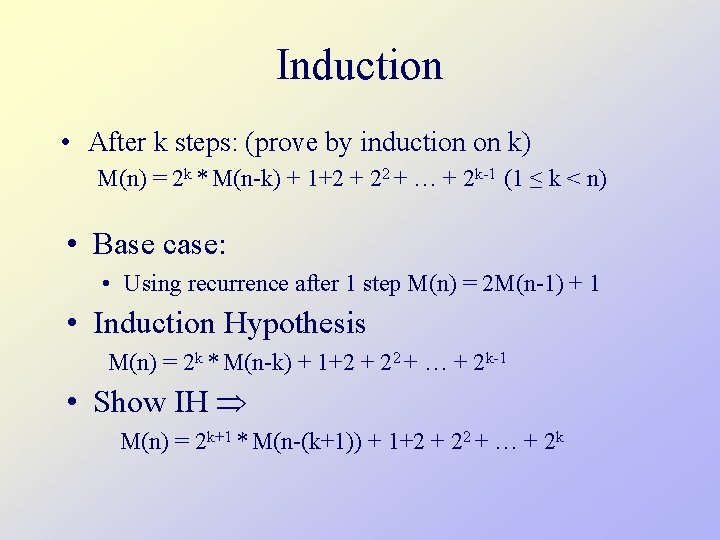Induction • After k steps: (prove by induction on k) M(n) = 2 k * M(n-k) + 1+2 + 22 + … + 2 k-1 (1 ≤ k < n) • Base case: • Using recurrence after 1 step M(n) = 2 M(n-1) + 1 • Induction Hypothesis M(n) = 2 k * M(n-k) + 1+2 + 22 + … + 2 k-1 • Show IH M(n) = 2 k+1 * M(n-(k+1)) + 1+2 + 22 + … + 2 k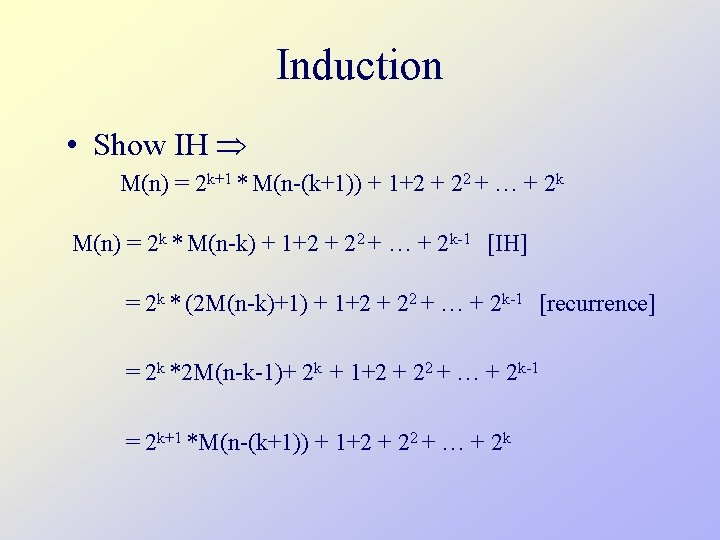Induction • Show IH M(n) = 2 k+1 * M(n-(k+1)) + 1+2 + 22 + … + 2 k M(n) = 2 k * M(n-k) + 1+2 + 22 + … + 2 k-1 [IH] = 2 k * (2 M(n-k)+1) + 1+2 + 22 + … + 2 k-1 [recurrence] = 2 k *2 M(n-k-1)+ 2 k + 1+2 + 22 + … + 2 k-1 = 2 k+1 *M(n-(k+1)) + 1+2 + 22 + … + 2 k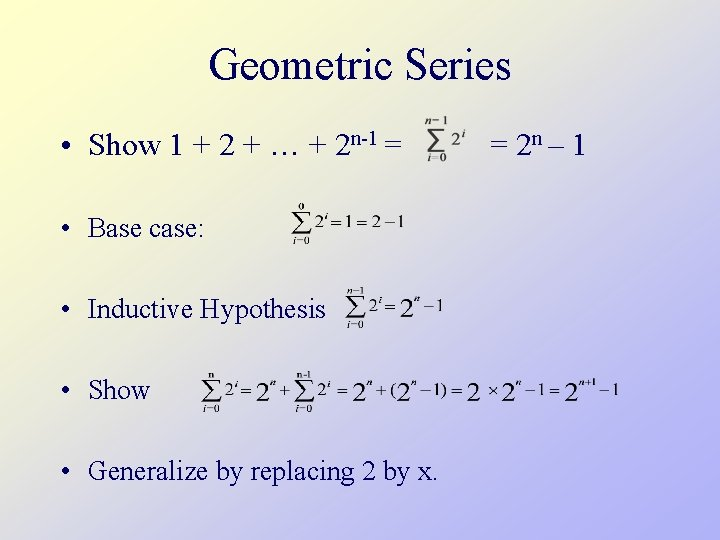Geometric Series • Show 1 + 2 + … + 2 n-1 = • Base case: • Inductive Hypothesis • Show • Generalize by replacing 2 by x. = 2 n – 1Worst Case • Is it possible to solve Hanoi in fewer than 2 n-1 moves? • Let M(n) be the fewest moves required to solve H(n, a, b, c) • Claim M(n) 2 M(n-1) + 1 [why? ] • Use induction to show M(n) 2 n-1Induction Examples Prove: • 1 + 3 + 5 + … + (2 n-1) = n 2 • 4 n < 2 n , n ≥ 5 • 7 | 8 n -1 • 2 n < n! , n ≥ 4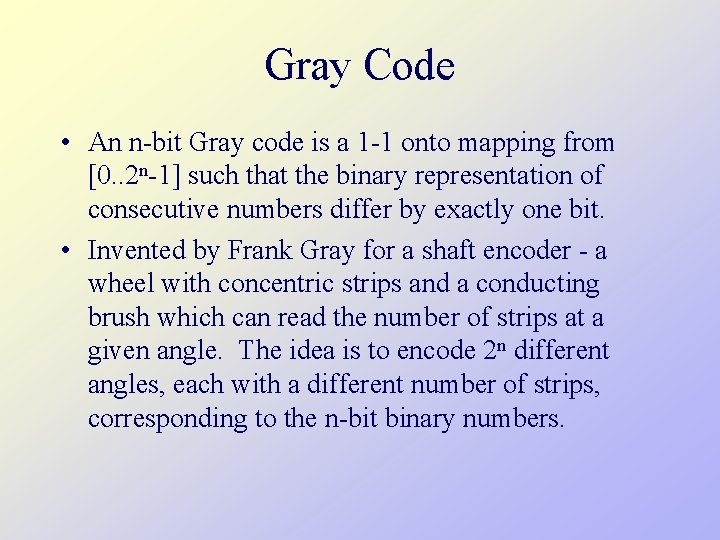Gray Code • An n-bit Gray code is a 1 -1 onto mapping from [0. . 2 n-1] such that the binary representation of consecutive numbers differ by exactly one bit. • Invented by Frank Gray for a shaft encoder - a wheel with concentric strips and a conducting brush which can read the number of strips at a given angle. The idea is to encode 2 n different angles, each with a different number of strips, corresponding to the n-bit binary numbers.Shaft Encoder (Counting Order) 010 011 100 001 000 111 101 110 Consecutive angles can have an abrupt change in the number of strips (bits) leading to potential detection errors.Shaft Encoder (Gray Code) 011 010 110 001 000 111 101 Since a Gray code is used, consecutive angles have only one change in the number of strips (bits).Binary-Reflected Gray Code • G 1 = [0, 1] • Gn = [0 Gn-1, 1 Gn-1], G reverse order complement leading bit • G 2 = [0 G 1, 1 G 1] = [00, 01, 10] • G 3 = [0 G 2, 1 G 2] = [000, 001, 010, 111, 100] • Use induction to prove that this is a Gray codeIterative Formula • Let Gn(i) be a function from [0, …, 2 n-1] • Gn(i) = i ^ (i >> 1) [exclusive or of i and i/2] – G 2(0) = 0, G 2(1) = 1, G 2(2) = 3, G 2(3) = 2 • Use induction to prove that the sequence Gn(i), i=0, …, 2 n-1 is a binary-reflected Gray code.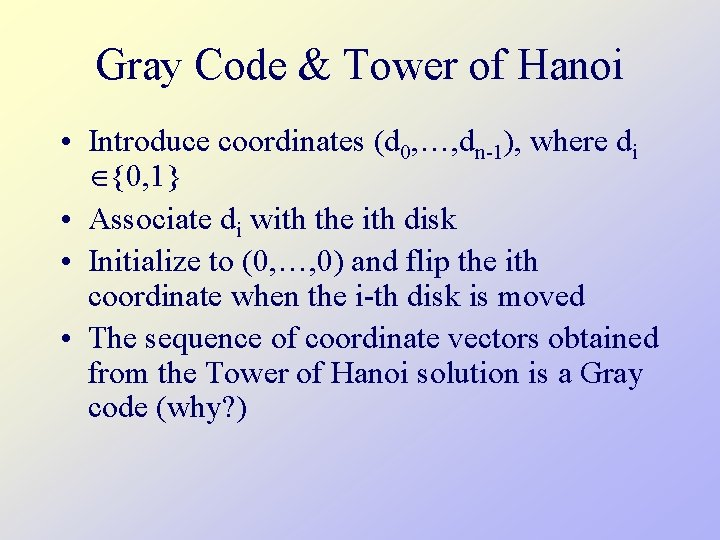Gray Code & Tower of Hanoi • Introduce coordinates (d 0, …, dn-1), where di {0, 1} • Associate di with the ith disk • Initialize to (0, …, 0) and flip the ith coordinate when the i-th disk is moved • The sequence of coordinate vectors obtained from the Tower of Hanoi solution is a Gray code (why? )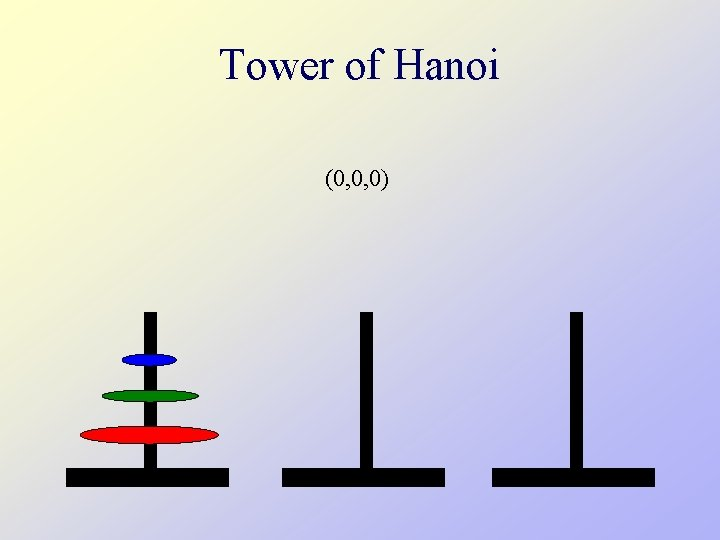Tower of Hanoi (0, 0, 0)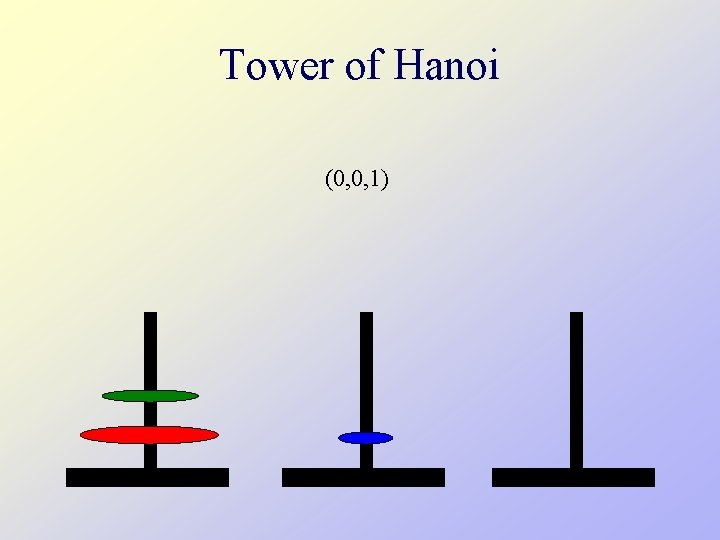Tower of Hanoi (0, 0, 1)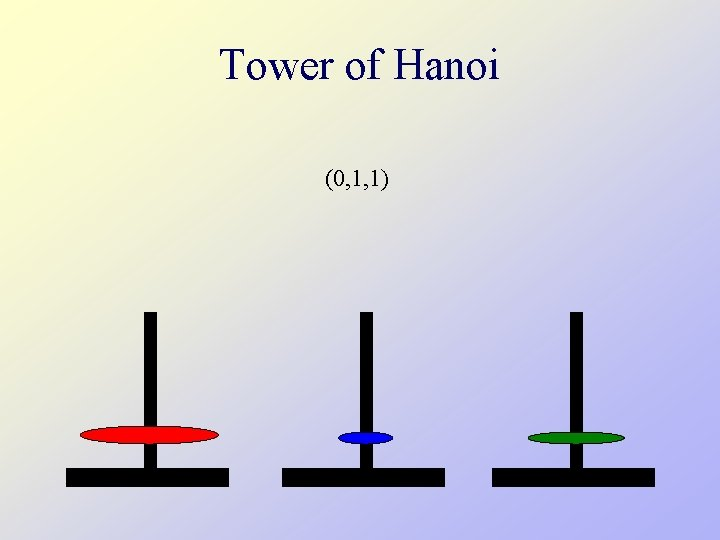Tower of Hanoi (0, 1, 1)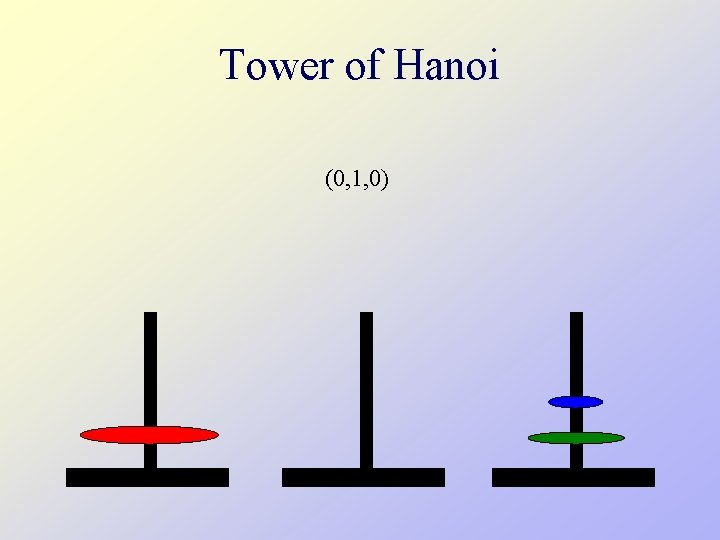Tower of Hanoi (0, 1, 0)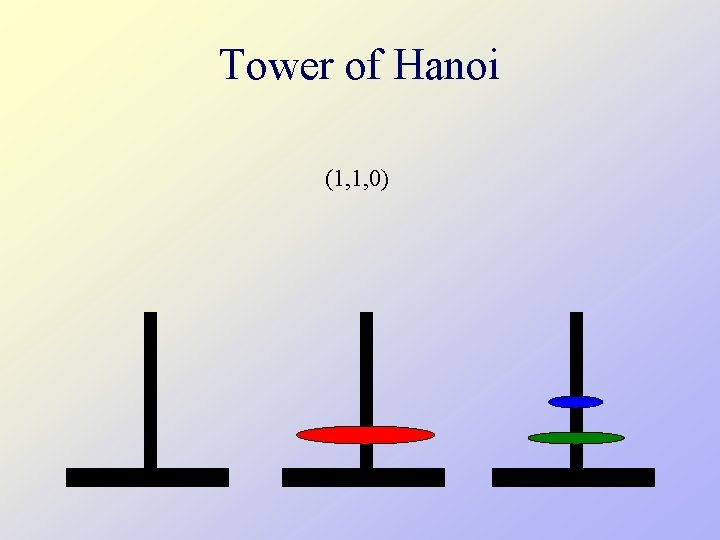Tower of Hanoi (1, 1, 0)Tower of Hanoi (1, 1, 1)Tower of Hanoi (1, 0, 1)Tower of Hanoi (1, 0, 0)Hypercube Graph (recursively defined) n-dimensional cube has 2 n nodes with each node connected to n vertices Binary labels of adjacent nodes differ in one bit 110 10 0 11 00 011 010 1 111 100 01 000 101 001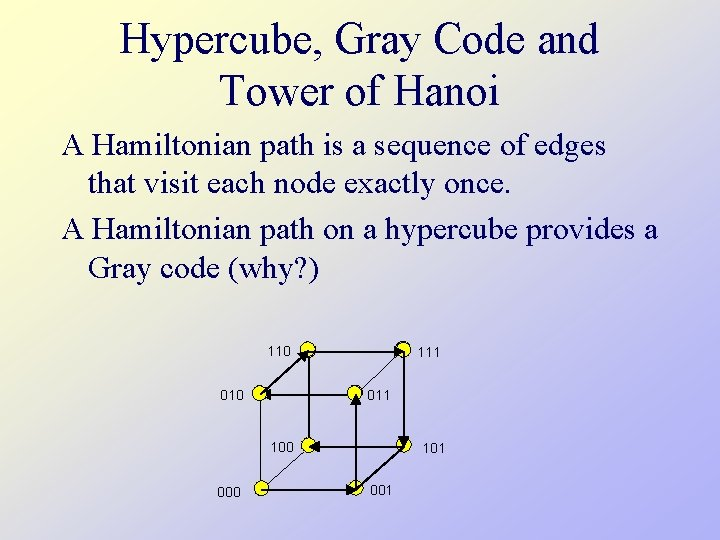Hypercube, Gray Code and Tower of Hanoi A Hamiltonian path is a sequence of edges that visit each node exactly once. A Hamiltonian path on a hypercube provides a Gray code (why? ) 110 111 010 100 000 101 001